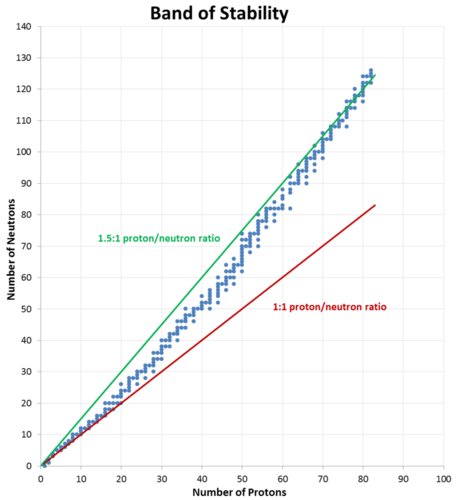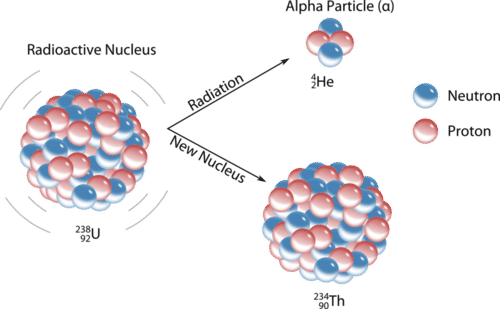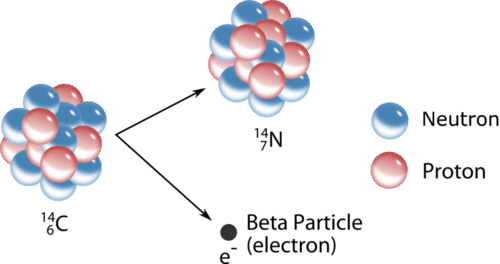•• Contributed by Allison Soult
• Senior Lecturer (Chemistry) at University of Kentucky

Learning Outcomes

• Explain how radioactivity involves a change in the nucleus of a radioisotope.
• Explain the characteristics of the forms of radiation.
• Describe and write equations for the primary types of radioactive decay.

Marie Curie (1867 - 1934) was a Polish scientist who pioneered research into nuclear radiation (Figure $$\PageIndex{1}$$). She was awarded the Nobel Prize in physics in 1903 along with her husband Pierre and Antoine Henri Becquerel for their work on radioactivity. She was awarded a second Nobel Prize in 1911, this time in chemistry, for her continued research on radioactive elements. In this lesson, you will learn about radioactivity, the reasons why certain elements and isotopes are radioactive, and the most common types of radioactive decay processes.Figure $$\PageIndex{1}$$: Marie Curie was one of the leading scientists in the field of radioactivity. She discovered two radioactive elements and was awarded two Nobel Prizes for her work.

Radioactivity was discovered quite by accident. In 1896, Henri Becquerel was studying the effect of certain uranium salts on photographic film plates. He believed that the salts had an effect on the film only when they had been exposed to sunlight. He accidentally found that uranium salts that had not been exposed to sunlight still had an effect on the photographic plates. The Curies, associates of Becquerel at the time, showed that the uranium was emitting a type of ray that interacted with the film. Marie Curie called this radioactivity. Radioactivity is the spontaneous breakdown of an atom's nucleus by the emission of particles and/or radiation. Radiation is the emission of energy through space in the form of particles and/or waves.

Nuclear reactions are very different from chemical reactions. In chemical reactions, atoms become more stable by participating in a transfer of electrons or by sharing electrons with other atoms. In nuclear reactions, it is the nucleus of the atom that gains stability by undergoing a change of some kind. Some elements have no stable isotopes, which means that any atom of that element is radioactive. For some other elements, only certain isotopes are radioactive. A radioisotope is an isotope of an element that is unstable and undergoes radioactive decay. The energies that are released in nuclear reactions are many orders of magnitude greater than the energies involved in chemical reactions. Unlike chemical reactions, nuclear reactions are not noticeably affected by changes in environmental conditions, such as temperature or pressure.

The discovery of radioactivity and its effects on the nuclei of elements disproved Dalton's assumption that atoms are indivisible. A nuclide is a term for an atom with a specific number of protons and neutrons in its nucleus. As we will see, when nuclides of one type emit radiation, they are changed into different nuclides. Radioactive decay is spontaneous and does not required an input of energy to occur. The stability of a particular nuclide depends on the composition of its nucleus, including the number of protons, the number of neutrons, and the proton-to-neutron ratio.

### The Band of Stability

Carbon-12, with six protons and six neutrons, is a stable nucleus, meaning that it does not spontaneously emit radioactivity. Carbon-14, with six protons and eight neutrons, is unstable and naturally radioactive. Among atoms with lower atomic numbers, the ideal ratio of neutrons to protons is approximately 1:1. As the atomic number increases, the stable neutron-proton ratio gradually increases to about 1.5:1 for the heaviest known elements. For example, lead-206 is a stable nucleus that contains 124 neutrons and 82 protons, a ratio of 1.51 to 1.

This observation is shown in the figure below. The band of stability is the range of stable nuclei on a graph that plots the number of neutrons in a nuclide against the number of protons. Known stable nuclides are shown with individual blue dots, while the 1:1 and 1.5:1 ratios are shown with a solid red line and a green line, respectively.Figure $$\PageIndex{2}$$: A graph of the number of neutrons in a nucleus as a function of the number of protons. Each known stable nucleus is represented by a blue dot. The ideal neutron to proton ratio changes from 1:1 for light nuclei to 1.5:1 for the heaviest nuclei.

It should be noted that just because a nucleus is "unstable" (able to undergo spontaneous radioactive decay) does not mean that it will rapidly decompose. For example, uranium-238 is unstable because it spontaneously decays over time, but if a sample of uranium-238 is allowed to sit for 1000 years, only $$0.0000155\%$$ of the sample will have decayed. However, other unstable nuclei, such as berkelium-243, will be almost completely gone (>$$99.9999\%$$ decayed) in less than a day.

Unstable nuclei spontaneously emit radiation in the form of particles and energy. This generally changes the number of protons and/or neutrons in the nucleus, resulting in a more stable nuclide. A nuclear reaction is a reaction that affects the nucleus of an atom. One type of a nuclear reaction is radioactive decay, a reaction in which a nucleus spontaneously disintegrates into a slightly lighter nucleus, accompanied by the emission of particles, energy, or both. An example is shown below, in which the nucleus of a polonium atom radioactively decays into a lead nucleus.

$\ce{^{210}_{84}Po} \rightarrow \ce{^{206}_{82}Pb} + \ce{^4_2He}$

Note that in a balanced nuclear equation, the sum of the atomic numbers (subscripts) and the sum of the mass numbers (superscripts) must be equal on both sides of the equation. Recall the notation system for isotopes, which shows both the atomic number and mass number along with the chemical symbol.Because the number of protons changes as a result of this nuclear reaction, the identity of the element changes. Transmutation is a change in the identity of a nucleus as a result of a change in the number of protons. There are several different types of naturally occurring radioactive decay, and we will examine each separately.

### Alpha Decay

An alpha particle $$\left( \alpha \right)$$ is a helium nucleus with two protons and two neutrons. Alpha particles are emitted during some types of radioactive decay. The net charge of an alpha particle is $$2+$$, and its mass is approximately $$4 \: \text{amu}$$. The symbol for an alpha particle in a nuclear equation is usually $$\ce{^4_2He}$$, though sometimes $$\alpha$$ is used. Alpha decay typically occurs for very heavy nuclei in which the nuclei are unstable due to large numbers of nucleons. For nuclei that undergo alpha decay, their stability is increased by the subtraction of two protons and two neutrons. For example, uranium-238 decays into thorium-234 by the emission of an alpha particle (see figure below).Figure $$\PageIndex{3}$$: The unstable uranium-238 nucleus spontaneously decays into a thorium-234 nucleus by emitting an alpha particle.

## Beta Decay

Nuclei above the band of stability are unstable because their neutron to proton ratio is too high. To decrease that ratio, a neutron in the nucleus is capable of turning into a proton and an electron. The electron is immediately ejected at a high speed from the nucleus. A beta particle $$\left( \beta \right)$$ is a high-speed electron emitted from the nucleus of an atom during some kinds of radioactive decay (see figure below). The symbol for a beta particle in an equation is either $$\beta$$ or $$\ce{^0_{-1}e}$$. Carbon-14 undergoes beta decay, transmutating into a nitrogen-14 nucleus.

$\ce{^{14}_6C} \rightarrow \ce{^{14}_7N} + \ce{^0_{-1}e}$

Note that beta decay increases the atomic number by one, but the mass number remains the same.Figure $$\PageIndex{4}$$: The beta decay of a carbon-14 nuclide involves the conversion of a neutron to a proton and an electron, with the electron being emitted from the nucleus.

### Positron Emission

Nuclei below the band of stability are unstable because their neutron to proton ratio is too low. One way to increase that ratio is for a proton in the nucleus to turn into a neutron and another particle called a positron. A positron is a particle with the same mass as an electron, but with a positive charge. Like the beta particle, a positron is immediately ejected from the nucleus upon its formation. The symbol for a positron in an equation is $$\ce{^0_{+1}e}$$. For example, potassium-38 emits a positron, becoming argon-38.

$\ce{^{38}_{19}K} \rightarrow \ce{^{38}_{18}Ar} + \ce{^0_1e}$

Positron emission decreases the atomic number by one, but the mass number remains the same.

### Electron Capture

An alternate way for a nuclide to increase its neutron to proton ratio is by a phenomenon called electron capture. In electron capture, an electron from an inner orbital is captured by the nucleus of the atom and combined with a proton to form a neutron. For example, silver-106 undergoes electron capture to become palladium-106.

$\ce{^{106}_{47}Ag} + \ce{^0_{-1}e} \rightarrow \ce{^{106}_{46}Pd}$

Note that the overall result of electron capture is identical to positron emission. The atomic number decreases by one while the mass number remains the same.

### Gamma Ray Emission

Gamma rays $$\left( \gamma \right)$$ are very high energy electromagnetic waves emitted from a nucleus. Gamma rays are emitted by a nucleus when nuclear particles undergo transitions between nuclear energy levels. This is analogous to the electromagnetic radiation emitted when excited electrons drop from higher to lower energy levels; the only difference is that nuclear transitions release much more energetic radiation. Gamma ray emission often accompanies the decay of a nuclide by other means.

$\ce{^{230}_{90}Th} \rightarrow \ce{^{226}_{88}Ra} + \ce{^4_2He} + \gamma$

The emission of gamma radiation has no effect on the atomic number or mass number of the products, but it reduces their energy.

The table below summarizes the main types of nuclear radiation, including charge, mass, symbol, and penetrating power. Penetrating power refers to the relative ability of the radiation to pass through common materials. Radiation with high penetrating power is potentially more dangerous because it can pass through skin and do cellular damage.

Table $$\PageIndex{1}$$ Summary of types of nuclear radiation.
Type Symbol Mass number Charge Penetration Power Shielding
Alpha particle $$\ce{^4_2He}$$ or $$\alpha$$ 4 $$2+$$ Low Paper, skin
Beta particle $$\ce{^0_{-1}e}$$ or $$\beta$$ 0 $$1-$$ Moderate Heavy cloth, plastic
Positron $$\ce{^0_1e}$$ or $$\beta^+$$ 0 $$1+$$ Moderate Heavy cloth, plastic
Gamma ray $$\gamma$$ or $$^0_0\gamma$$ 0 0 High Lead, concrete
Neutron $$\ce{^1_0n}$$ 1 0 High Water, leadFigure $$\PageIndex{5}$$: Infographic showing the different properties of radiation. "A Guide to Different Types of Radiation" is by Compound Interest is licensed under CC BY NC ND 4.0.

## Supplemental Resources

• Atomic structure review: www.sciencegeek.net/Chemistry...t1Numbers2.htm
• Balancing Nuclear equations: www.sciencegeek.net/Chemistry...rEquations.htm
• Nuclear decay: www.sciencegeek.net/Chemistry...cleardecay.htm